# Effective Brainstorming: How to Harvest Constructive Creativity | Seattle Interactive Conference 2018

28 Oct 2018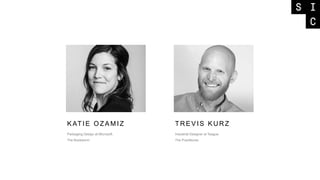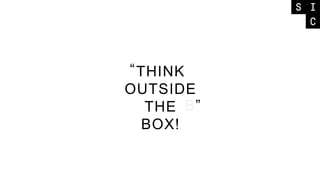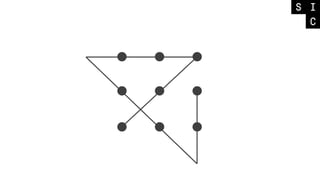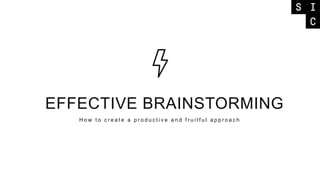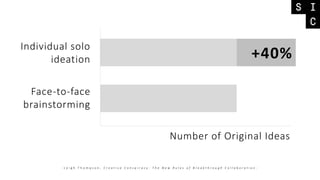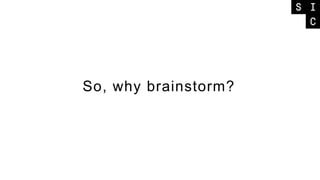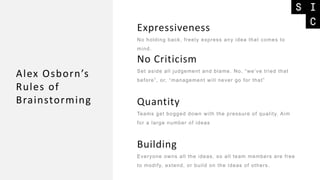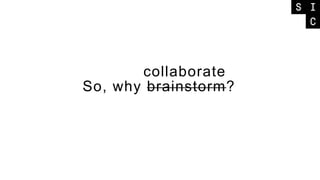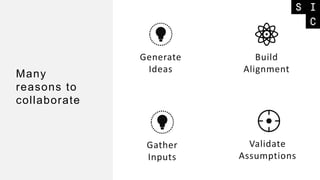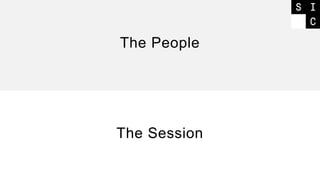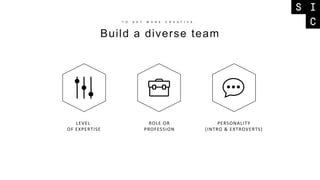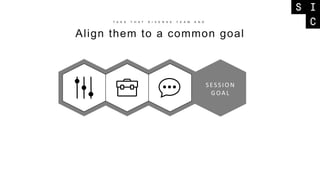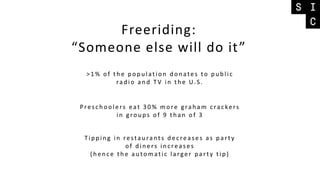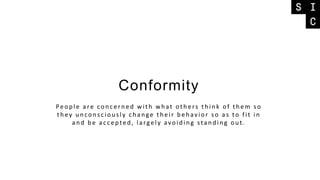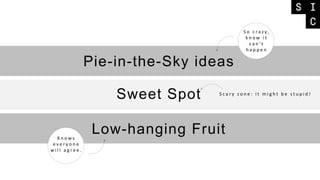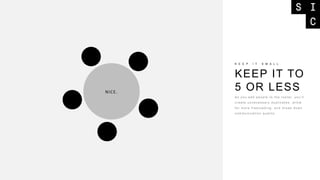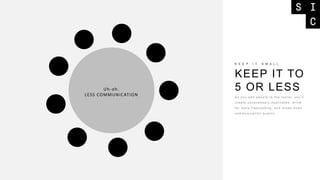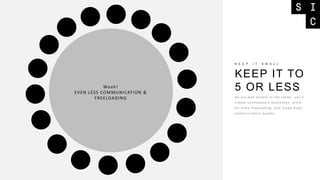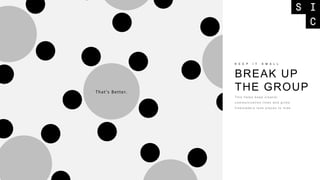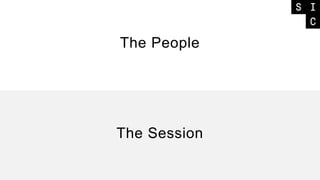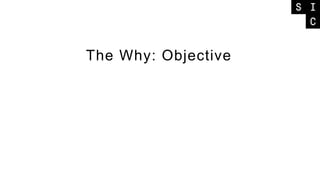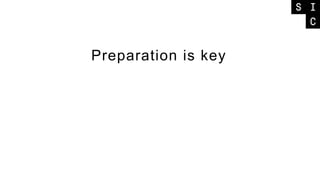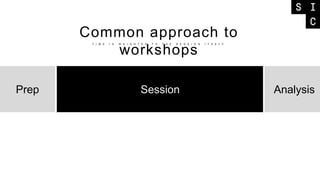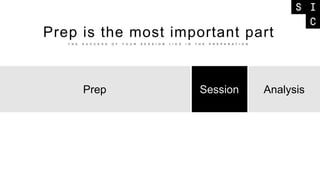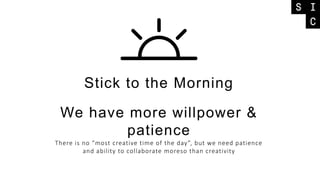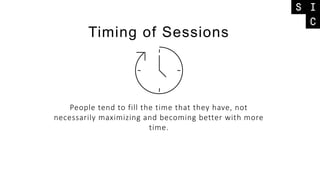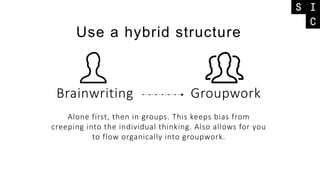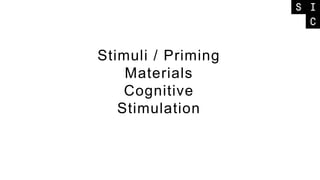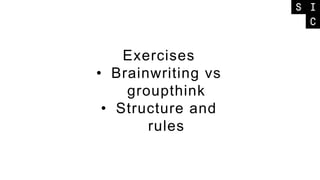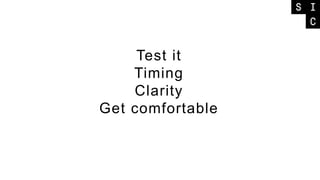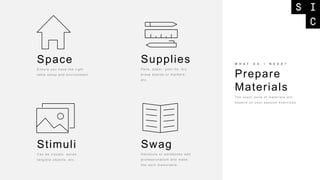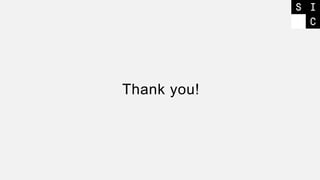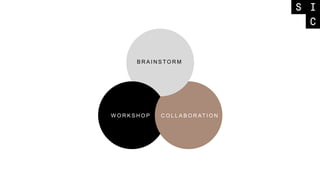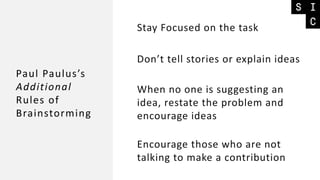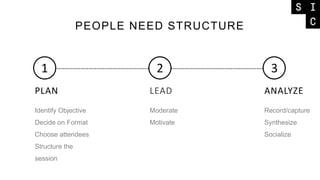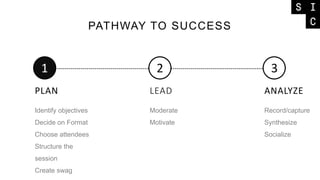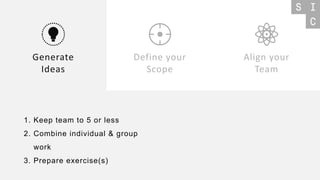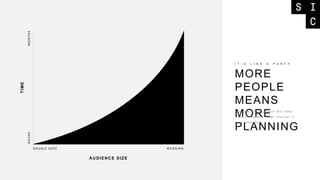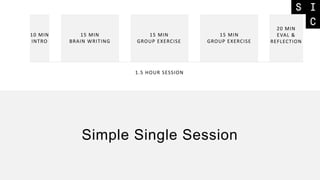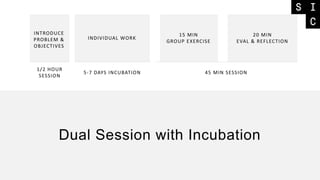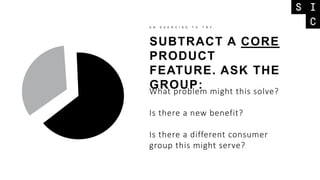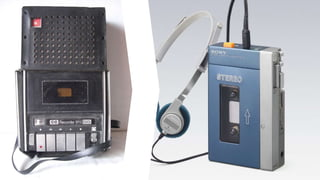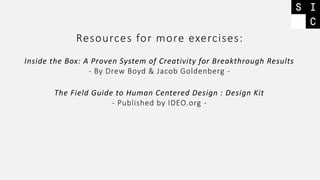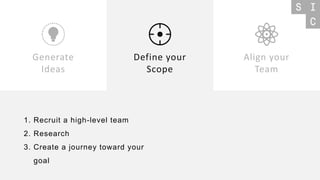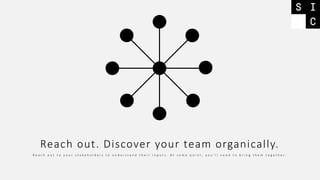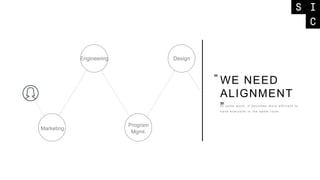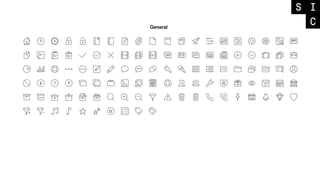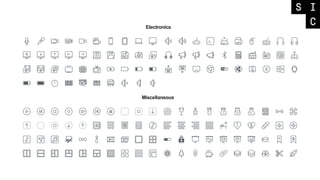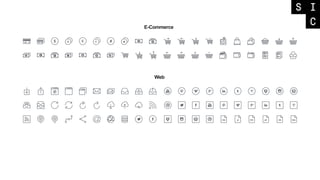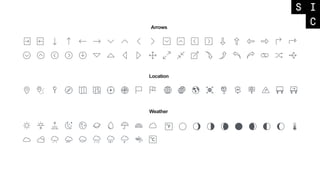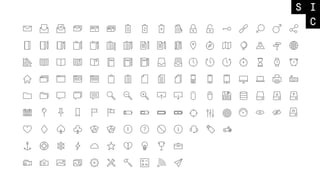1 sur 55

### Effective Brainstorming: How to Harvest Constructive Creativity | Seattle Interactive Conference 2018

• 1. Packaging Design at Microsoft. The Bookworm KATIE OZAMIZ Industrial Designer at Teague. The Practitioner. TREVIS KURZ
• 5. EFFECTIVE BRAINSTORMING H o w t o c r e a t e a p r o d u c t i v e a n d f r u i t f u l a p p r o a c h
• 6. Number of Original Ideas Individual solo ideation Face-to-face brainstorming - L e i g h T h o m p s o n , C r e a t i v e C o n s p i r a c y : T h e N e w R u l e s o f B r e a k t h r o u g h C o l l a b o r a t i o n - +40%
• 8. Expressiveness Alex Osborn’s Rules of Brainstorming No holding back, freely express any idea that comes to mind. No Criticism Set aside all judgement and blame. No, “we’ve tried that before”, or, “management will never go for that” Quantity Teams get bogged down with the pressure of quality. Aim for a large number of ideas Building Everyone owns all the ideas, so all team members are free to modify, extend, or build on the ideas of others.
• 12. Build a diverse team T O G E T M O R E C R E A T I V E , LEVEL OF EXPERTISE ROLE OR PROFESSION PERSONALITY (INTRO & EXTROVERTS)
• 13. Align them to a common goal T A K E T H A T D I V E R S E T E A M A N D SESSION GOAL
• 14. Freeriding: “Someone else will do it” >1% o f the po pulatio n do nates to public radio and TV in the U.S. Prescho o lers eat 30% mo re gra ha m cra ckers in gro ups o f 9 tha n o f 3 Tipping in resta ura nts decrea ses a s pa rty o f diners increa ses (hence the a uto matic la rger pa rty tip)
• 15. Conformity Peo ple a re co ncerned with what o thers think o f them so they unco nscio usly cha nge their behavio r so a s to fit in a nd be a ccepted, la rgely avo iding sta nding o ut.
• 16. Peo ple a re co ncerned with what o thers think o f them so they unco nscio usly cha nge their behavio r so a s to fit in a nd be a ccepted, la rgely avo iding sta nding o ut. Pie-in-the-Sky ideas Low-hanging Fruit Sweet Spot S o c r a z y, k n o w i t c a n ’ t h a p p e n K n o w s e v e r y o n e w i l l a g r e e . S c a r y z o n e : i t m i g h t b e s t u p i d !
• 17. KEEP IT TO 5 OR LESS K E E P I T S M A L L A s y o u a d d p e o p l e t o t h e r o s t e r, y o u ’ l l c r e a t e u n n e c e s s a r y d u p l i c a t e s , a l l o w f o r m o r e f r e e l o a d i n g , a n d b r e a k d o wn c o m m u n i c a t i o n q u a l i t y NICE.
• 18. KEEP IT TO 5 OR LESS K E E P I T S M A L L A s y o u a d d p e o p l e t o t h e r o s t e r, y o u ’ l l c r e a t e u n n e c e s s a r y d u p l i c a t e s , a l l o w f o r m o r e f r e e l o a d i n g , a n d b r e a k d o wn c o m m u n i c a t i o n q u a l i t y Uh-oh. LESS COMMUNICATION
• 19. KEEP IT TO 5 OR LESS K E E P I T S M A L L A s y o u a d d p e o p l e t o t h e r o s t e r, y o u ’ l l c r e a t e u n n e c e s s a r y d u p l i c a t e s , a l l o w f o r m o r e f r e e l o a d i n g , a n d b r e a k d o wn c o m m u n i c a t i o n q u a l i t y Woah! EVEN LESS COMMUNICATION & FREELOADING
• 20. BREAK UP THE GROUP K E E P I T S M A L L T h i s h e l p s k e e p c l e a n e r c o m m u n i c a t i o n l i n e s a n d g i v e s f r e e l o a d e r s l e s s p l a c e s t o h i d e . That’s Better.
• 24. SessionPrep Analysis T I M E I S W E I G H T E D T O T H E S E S S I O N I T S E L F Common approach to workshops
• 25. SessionPrep Analysis T H E S U C C E S S O F Y O U R S E S S I O N L I E S I N T H E P R E P A R A T I O N Prep is the most important part
• 26. Stick to the Morning We have more willpower & patience There is no “most creative time of the day”, but we need patience and ability to collaborate moreso than creativity
• 27. Timing of Sessions People tend to fill the time that they have, not necessarily maximizing and becoming better with more time.
• 28. Use a hybrid structure Brainwriting Groupwork Alone first, then in groups. This keeps bias from creeping into the individual thinking. Also allows for you to flow organically into groupwork.
• 32. Prepare Materials W H A T D O I N E E D ? T h e e x a c t s u i t e o f m a t e r i a l s wi l l d e p e n d o n y o u r s e s s i o n e x e r c i s e s . Stimuli C a n b e v i s u a l s , wo r d s , t a n g i b l e o b j e c t s , e t c . Supplies P e n s , p a p e r, p o s t - i t s , d r y e r a s e b o a r d s o r m a r k e r s , e t c . Space E n s u r e y o u h a v e t h e r i g h t t a b l e s e t u p a n d e n v i r o n m e n t Swag H a n d o u t s o r wo r k b o o k s a d d p r o f e s s i o n a l i s m a n d m a k e t h e wo r k m e m o r a b l e .
• 35. B R A I N S T O R M W O R K S H O P C O L L A B O R AT I O N
• 36. Stay Focused on the task Paul Paulus’s Additional Rules of Brainstorming Don’t tell stories or explain ideas When no one is suggesting an idea, restate the problem and encourage ideas Encourage those who are not talking to make a contribution
• 37. LEADPLAN ANALYZE 1 2 3 PEOPLE NEED STRUCTURE Identify Objective Decide on Format Choose attendees Structure the session Moderate Motivate Record/capture Synthesize Socialize
• 38. LEADPLAN ANALYZE 1 2 3 PATHWAY TO SUCCESS Identify objectives Decide on Format Choose attendees Structure the session Create swag Moderate Motivate Record/capture Synthesize Socialize
• 39. Align your Team Define your Scope Generate Ideas 1. Keep team to 5 or less 2. Combine individual & group work 3. Prepare exercise(s)
• 40. MORE PEOPLE MEANS MORE PLANNING I T ’ S L I K E A P A R T Y A n d c l i e n t - f a c i n g wo r k wi l l n e e d m o r e p r e p a r a t i o n t h a n i n t e r n a l o r c o r e t e a m wo r k . TIME DO UBLE DATE AUDIENCE SIZE W E DDING HOURSMONTHS
• 41. 1.5 HOUR SESSION 15 MIN BRAIN WRITING 10 MIN INTRO 15 MIN GROUP EXERCISE 20 MIN EVAL & REFLECTION 15 MIN GROUP EXERCISE Simple Single Session
• 42. Dual Session with Incubation 1/2 HOUR SESSION 15 MIN GROUP EXERCISE 20 MIN EVAL & REFLECTION INTRODUCE PROBLEM & OBJECTIVES 5-7 DAYS INCUBATION 45 MIN SESSION INDIVIDUAL WORK
• 43. SUBTRACT A CORE PRODUCT FEATURE. ASK THE GROUP: A N E X E R C I S E T O T R Y : What problem might this solve? Is there a new benefit? Is there a different consumer group this might serve?
• 45. Resources for more exercises: Inside the Box: A Proven System of Creativity for Breakthrough Results - By Drew Boyd & Jacob Goldenberg - The Field Guide to Human Centered Design : Design Kit - Published by IDEO.org -
• 46. Generate Ideas Align your Team 1. Recruit a high-level team 2. Research 3. Create a journey toward your goal Define your Scope
• 47. Reach out. Discover your team organically. R e a c h o u t t o y o u r s t a k e h o l d e r s t o u n d e r s t a n d t h e i r i n p u t s . A t s o m e p o i n t , y o u ’ l l n e e d t o b r i n g t h e m t o g e t h e r .
• 48. WE NEED ALIGNMENT ”A t s o m e p o i n t , i t b e c o m e s m o r e e ff i c i e n t t o h a v e e v e r y o n e i n t h e s a m e r o o m . Marketing Engineering Program Mgmt. Design “
• 49. WHAT DO YOU NEED? F I R S T D E F I N E : T h i s g i v e s u s o u r d e s t i n a t i o n 1. DEFINE DESIRED OUTPUT
• 50. HOW WILL WE GET THERE? N E X T T H I N K A B O U T T h i n k a b o u t e x e r c i s e s t h a t wi l l g e t wh a t y o u n e e d f r o m y o u r a u d i e n c e .1. DEFINE DESIRED OUTPUT 2. BUILD A PATH

### Notes de l'éditeur

1. Only 20% were able to figure this out. Went viral (media & word of mouth). Years later, 2 other research teams ran the same experiment but with one group being told the “trick” in advance (to extend beyond the box of the dots). Only 25% got it right (basically statistically insignificant)
2. Only 20% were able to figure this out. Went viral (media & word of mouth). Years later, 2 other research teams ran the same experiment but with one group being told the “trick” in advance (to extend beyond the box of the dots). Only 25% got it right (basically statistically insignificant)